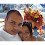## Sunday, October 9, 2011

### Playing sound source code in C++

This source code is not for playing songs or sound clips from your hard drive only to play  sound by using windows function Beep(int dwFreq [in],int dwDuration [in]) .
Parameters are
dwFreq [in]
The frequency of the sound, in hertz. This parameter must be in the range 37 through 32,767                      (0x25 through 0x7FFF)
dwDuration [in]
The duration of the sound, in milliseconds.
If the function succeeds, the return value is nonzero.If the function fails, the return value is zero. To get extended error information, call GetLastError.
This source code play the music “twinkle twinkle little star” using windows command Beep.Here is the source code copy it and compile and run in code::blocks IDE.

` `

`#include <iostream>`
`#include <windows.h>`
`using namespace std;`
`//twinkle twinkle`
`//CC GG AA G`
`//FF EE DD C`
`//GG FF EE D`
`//GG FF EE D`
`//CC GG AA G`
`//FF EE DD C`
`void playnote (char g, float l)`
`{`

`char n = g;`

`if (n == 'A'||'a'){`
`Beep(2750,l*1000);`
`cout <<n;`
`}`
`else if(n == 'B'||'b'){`
`Beep(3087,l*1000);`
`cout << n;`
`}`
`else if(n == 'C'||'c'){`
`Beep(1637,l*1000);`
`cout << n;`
`}`
`else if(n == 'D'||'d'){`
`Beep(1835,l*1000);`
`cout << n;`
`}`
`else if(n == 'E'||'e'){`
`Beep(2060,l*1000);`
`cout << n;`
`}`
`else if(n == 'F'||'f'){`
`Beep(2183,l*1000);`
`cout << n;`
`}`
`else if(n == 'G'||'g'){`
`Beep(2450,l*1000);`
`cout << n;`
`}`
`}`
` `
`int main() {`

`playnote('C', 0.4);`
`playnote('C', 0.4);`
`playnote('G', 0.4);`
`playnote('G', 0.4);`
`playnote('A', 0.4);`
`playnote('A', 0.4);`
`playnote('G', 0.4);`
`Sleep(400);`
`cout<<endl;`
`playnote('F', 0.4);`
`playnote('F', 0.4);`
`playnote('E', 0.4);`
`playnote('E', 0.4);`
`playnote('D', 0.4);`
`playnote('D', 0.4);`
`playnote('C', 0.4);`
`Sleep(400);`
`cout<<endl;`
`playnote('G', 0.4);`
`playnote('G', 0.4);`
`playnote('F', 0.4);`
`playnote('F', 0.4);`
`playnote('E', 0.4);`
`playnote('D', 0.4);`
`playnote('G', 0.4);`
`Sleep(400);`
`cout<<endl;`
`playnote('G', 0.4);`
`playnote('F', 0.4);`
`playnote('F', 0.4);`
`playnote('E', 0.4);`
`playnote('D', 0.4);`
`playnote('C', 0.4);`
`playnote('C', 0.4);`
`Sleep(400);`
`cout<<endl;`
`playnote('G', 0.4);`
`playnote('G', 0.4);`
`playnote('A', 0.4);`
`playnote('A', 0.4);`
`playnote('G', 0.4);`
`playnote('F', 0.4);`
`playnote('F', 0.4);`
`Sleep(400);`
`cout<<endl;`
`playnote('E', 0.4);`
`playnote('E', 0.4);`
`playnote('D', 0.4);`
`playnote('D', 0.4);`
`playnote('C', 0.4);`
`cout<<endl;`
`cout << "thanks for listening <3";`

`return 0;`
` `
`}`

1.Sorry I'm brand new to C++ & codeblocks. Is the beep supposed to be the same tone for every beep it makes? Thank you!

2.how about introduction, pseudocode and flow chart ?

3.The reason why the tune is the same all the way through is, it always plays A (or a) to fix it,
if (n == 'A'||'a'){
needs to be changed to
if ((n == 'A')||(n == 'a')){
and the same to the other if B to G lines, but most likely you have worked that out already.

From Eric Richards

1.thanks!! it works!!